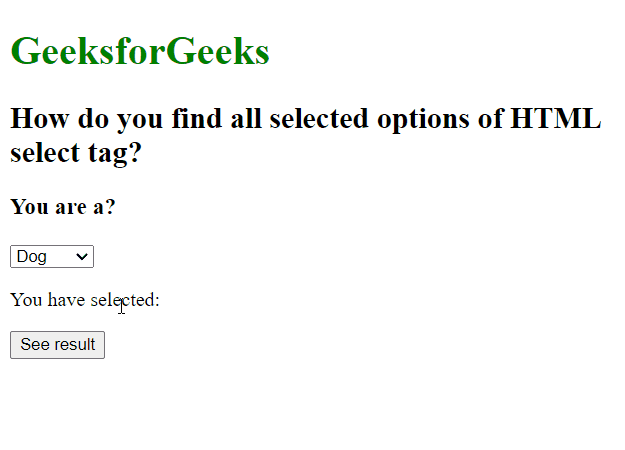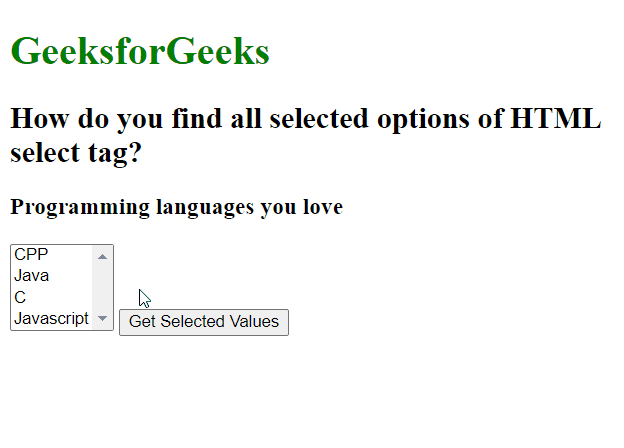GFG App
Open AppBrowser
Continue

# How to find all selected options of HTML select tag ?

In this article, we will learn how to find all selected options of the HTML <select> tag. This might be needed in cases where you might have been given some multiple choice-based exams where you need to choose the correct option for getting marks. To achieve the same, we first have to find the selected option and match it with the correct option. we can build it very easily with the help of JQuery.

Here we are going to see two cases:

1. When you want to get the selected option value from a single-select drop-down.
2. When you want to get selected options values from a multi-select drop-down.

Example 1: Find a single selected option from HTML select tag.

## HTML

 `` `<``html` `lang``=``"en"``>`   `<``head``>` `    ``<``script` `src``=` `"https://code.jquery.com/jquery-3.6.0.min.js"``>` `    ``` ``   `<``body``>` `    ``<``h1` `style``=``"color: green;"``>GeeksforGeeks` `    ``<``h2``>How do you find all selected options` `        ``of HTML select tag?` `    ``<``h3``>You are a?` `    ``<``select` `id``=``"animals"``>` `        ``<``option` `value``=``"dog"``>Dog` `        ``<``option` `value``=``"cat"``>Cat` `        ``<``option` `value``=``"lion"``>Rabbit` `        ``<``option` `value``=``"human"``>Human` `    ``` `    `  `<``p``>` `        ``You have selected: ` `        ``<``span` `id``=``"selected"``>` `    ```   `    ``<``p` `id``=``"correct"``>`     `    ``<``button` `id``=``"submit"``>See result` `    ``<``script``>` `        ``\$(document).ready(function () {` `            ``const ans = "The correct answer is: Human";` `            ``let chosen = "Your answer is: ";` `            ``\$("#submit").click(function () {` `                ``const vals =` `                      ``\$("#animals").find(` `                        ``"option:selected"` `                      ``).text();`   `                ``\$("#correct").text(ans);` `                ``\$("#selected").text(vals);` `            ``});` `        ``});` `    ``` ``   ``

Output:In some conditions, we need only need a single option to get selected like above where you need to select whether you are a dog, cat, rabbit, or human and of course, if you are reading this post then you are a human. Hence other options become insignificant. In such conditions we can either use \$(“select”) .find(“option:selected”) or simply \$(“option:selected”). In both the methods “option:selected” is a must which gives us the first selected option.

Note: It is suggested to use \$(“select”) .find(“option:selected”) because using this you can select options from a specific select tag if multiple select tags are there.

Example 2: Get multiple selected options from an HTML select tag.

## HTML

 `` `<``html` `lang``=``"en"``>`   `<``head``>` `    ``<``script` `src``=` `"https://code.jquery.com/jquery-3.6.0.min.js"``>` `    ``` ``   `<``body``>` `    ``<``h1` `style``=``"color: green;"``>GeeksforGeeks` `    ``<``h2``>How do you find all selected options` `        ``of HTML select tag?` `    ``<``h3``>Programming languages you love` `    ``<``select` `id``=``"languages"` `multiple``=``"multiple"``>` `        ``<``option` `value``=``"cpp"``>CPP` `        ``<``option` `value``=``"java"``>Java` `        ``<``option` `value``=``"c"``>C` `        ``<``option` `value``=``"js"``>Javascript` `    ``` `    ``<``button` `id``=``"submit"``>Get Selected Values` `    ``<``script``>` `        ``\$(document).ready(function () {` `            ``\$("#submit").click(function () {` `                ``const opts = \$(` `                  ``"#languages option:selected"` `                ``).map(function () {` `                    ``return this.value;` `                ``}).get().join(",");` `                ``alert("Selected options are: " + opts);` `            ``});` `        ``});` `    ``` ``   ``

Output:My Personal Notes arrow_drop_up